## The Method of Undetermined Coefficients

In order to give the complete solution of a nonhomogeneous linear differential equation, Theorem B says that a particular solution must be added to the general solution of the corresponding homogeneous equation.

If the nonhomogeneous term dx) in the general second‐order nonhomogeneous differential equation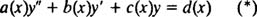is of a certain special type, then the method of undetermined coefficientscan be used to obtain a particular solution. The special functions that can be handled by this method are those that have a finite family of derivatives, that is, functions with the property that all their derivatives can be written in terms of just a finite number of other functions.

For example, consider the function d = sin x. Its derivatives are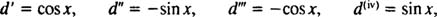and the cycle repeats. Notice that all derivatives of d can be written in terms of a finite number of functions. [In this case, they are sin x and cos x, and the set {sin x, cos x} is called the family (of derivatives) of d = sin x.] This is the criterion that describes those nonhomogeneous terms dx) that make equation (*) susceptible to the method of undetermined coefficients: d must have a finite family.

Here's an example of a function that does not have a finite family of derivatives: d = tan x. Its first four derivatives areNotice that the nth derivative ( n ≥ 1) contains a term involving tan n‐1 x, so as higher and higher derivatives are taken, each one will contain a higher and higher power of tan x, so there is no way that all derivatives can be written in terms of a finite number of functions. The method of undetermined coefficients could not be applied if the nonhomogeneous term in (*) were d = tan x. So just what are the functions dx) whose derivative families are finite? See Table 1.Example 1: If dx) = 5 x 2, then its family is { x 2x, 1}. Note that any numerical coefficients (such as the 5 in this case) are ignored when determining a function's family.

Example 2: Since the function dx) = x sin 2 x is the product of x and sin 2 x, the family of dx) would consist of all products of the family members of the functions x and sin 2 x. That is,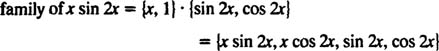Linear combinations of n functions . A linear combination of two functions y 1 and y 2 was defined to be any expression of the formwhere c 1 and c 2 are constants. In general, a lineral, a linear combination of n functions y 1 y 2,…, n is any expression of the form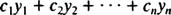where c 1,…, n are contants. Using this terminology, the nonhomogeneous terms dx) which the method of undetermined coefficients is designed to handle are those for which every derivative can be written as a linear combination of the members of a given finite family of functions.

The central idea of the method of undetermined coefficients is this: Form the most general linear combination of the functions in the family of the nonhomogeneous term dx), substitute this expression into the given nonhomogeneous differential equation, and solve for the coefficients of the linear combination.

Example 3: Find a particular solution of the differential equation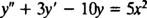As noted in Example 1, the family of d = 5 x 2 is { x 2x, 1}; therefore, the most general linear combination of the functions in the family is y = Ax 2 + Bx + C (where AB, and C are the undetermined coefficients). Substituting this into the given differential equation givesNow, combinbing like terms yieldsIn order for this last equation to be an identity, the coefficients of like powers of x on both sides of the equation must be equated. That is, AB, and C must be chosen so thatThe first equation immediately gives. Substituting this into the second equation gives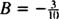, and finally, substituting both of these values into the last equation yields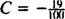. Therefore, a particular solution of the given differential equation is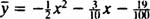Example 4: Find a particular solution (and the complete solution) of the differential equationSince the family of d = sin x is {sin x, cos x}, the most general linear combination of the functions in the family is y = A sin x + B cos x (where A and B are the undetermined coefficients). Substituting this into the given differential equation gives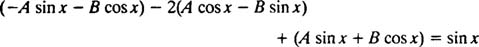Now, combining like terms and simplifying yieldsIn order for this last equation to be an identity, the coefficients A and B must be chosen so thatThese equations immediately imply A = 0 and B = ½. A particular solution of the given differential equation is thereforeAccording to Theorem B, combining this y with the result of Example 12 yields the complete solution of the given nonhomogeneous differential equation: y = c 1 e x c 2 xe x + ½ cos x.

Example 5: Find a particular solution (and the complete solution) of the differential equation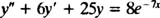Since the family of d = 8 e −7 x is just { e −7 x }, the most general linear combination of the functions in the family is simply y = Ae −7 x (where A is the undetermined coefficient). Substituting this into the given differential equation gives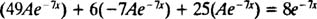Simplifying yieldsIn order for this last equation to be an identity, the coefficient A must be chosen so thatwhich immediately gives A = ¼. A particular solution of the given differential equation is therefore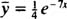and then, according to Theorem B, combining y with the result of Example 13 gives the complete solution of the nonhomogeneous differential equation: y = e −3 x c 1 cos 4 x + c 2 sin 4 x) + ¼ e −7 x .

Example 6: Find the solution of the IVP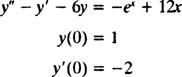The first step is to obtain the general solution of the corresponding homogeneous equation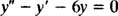Since the auxiliary polynomial equation has distinct real roots,the general solution of the corresponding homogeneous equation is y h c 1 e − x c 2 e x

Now, since the nonhomogeneous term dx) is a (finite) sum of functions from Table 1, the family of dx) is the union of the families of the individual functions. That is, since the family of − e x is { e x }, and the family of 12x is { x, 1},The most general linear combination of the functions in the family of d = − e x + 12 x is therefore y = Ae x Bx + C (where AB, and C are the undetermined coefficients). Substituting this into the given differential equation givesCombining like terms and simplifying yieldsIn order for this last equation to be an identity, the coefficients AB, and C must be chosen so thatThe first two equations immediately give A = ⅙ and B = −2, whereupon the third implies C = ⅓. A particular solution of the given differential equation is therefore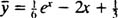According to Theorem B, then, combining this y with y h gives the complete solution of the nonhomogeneous differential equation: y = c 1 e −2 x c 2 e x + ⅙ e x –2 x + ⅓. Now, to apply the initial conditions and evaluate the parameters c 1 and c 2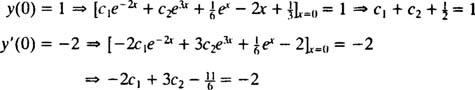Solving these last two equations yields c 1 = ⅓ and c 2 = ⅙. Therefore, the desired solution of the IVP is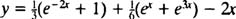Now that the basic process of the method of undetermined coefficients has been illustrated, it is time to mention that is isn't always this straightforward. A problem arises if a member of a family of the nonhomogeneous term happens to be a solution of the corresponding homogeneous equation. In this case, that family must be modified before the general linear combination can be substituted into the original nonhomogeneous differential equation to solve for the undetermined coefficients. The specific modification procedure will be introduced through the following alteration of Example 6.

Example 7: Find the complete solution of the differential equationThe general solution of the corresponding homogeneous equation was obtained in Example 6: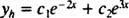Note carefully that the family { e x } of the nonhomogeneous term d = 10 e x contains a solution of the corresponding homogeneous equation (take c 1 = 0 and c 2 = 1 in the expression for y h ). The “offending” family is modified as follows: Multiply each member of the family by x and try again.Since the modified family no longer contains a solution of the corresponding homogeneous equation, the method of undetermined coefficients can now proceed. (If xe x had been again a solution of the corresponding homogeneous equation, you would perform the modification procedure once more: Multiply each member of the family by x and try again.) Therefore, substituting y = Axe x into the given nonhomogeneous differential equation yields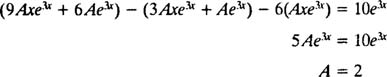This calculation implies that y = 2 xe x is a particular solution of the nonhomogeneous equation, so combining this with y h gives the complete solution: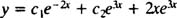Example 8: Find the complete solution of the differential equation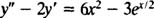First, obtain the general solution of the corresponding homogeneous equation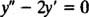Since the auxiliary polynomial equation has distinct real roots,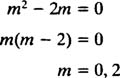the general solution of the corresponding homogeneous equation isThe family for the 6 x 2 term is { x 2x, 1}, and the family for the −3 e x/2 term is simply { e x/2 }. This latter family does not contain a solution of the corresponding homogeneous equation, but the family { x 2x, 1} does(it contains the constant function 1, which matches y h when c 1 = 1 and c 2 = 0). This entire family (not just the “offending” member) must therefore be modified:The family that will be used to construct the linear combination y is now the union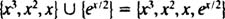This implies that y = Ax 3 + Bx 2 + Cx + De x/2 (where ABC, and D are the undetermined coefficients) should be substituted into the given nonhomogeneous differential equation. Doing so yields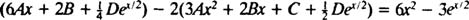which after combining like terms reads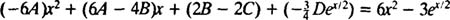In order for this last equation to be an identity, the coefficients ABC, and D must be chosen so that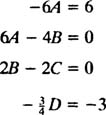These equations determine the values of the coefficients: A = −1, B = C =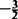, and D = 4. Therefore, a particular solution of the given differential equation isAccording to Theorem B, then, combining this y with y h gives the complete solution of the nonhomogeneous differential equation: y = c 1 + c 2 e x – x 3x 2x + 4 e x/2

Example 9: Find the complete solution of the equation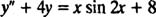First, obtain the general solution of the corresponding homogeneous equation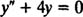Since the auxiliary polynomial equation has distinct conjugate complex roots,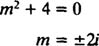the general solution of the corresponding homogeneous equation is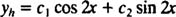Example 2 showed that the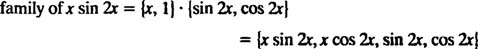Note that this family contains sin 2 x and cos 2 x, which are solutions of the corresponding homogeneous equation. Therefore, this entire family must be modified:None of the members of this family are solutions of the corresponding homogeneous equation, so the solution can now proceed as usual. Since the family of the constant term is simply {1}, the family used to construct y is the union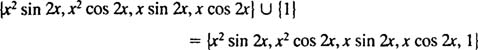This implies that y = Ax 2 sin 2 x + Bx 2 cos 2 x + Cx sin 2 x + Dx cos 2 x + E (where ABCD, and E are the undermined coefficients) should be substitueted into the given nonhomogeneous differential equation y″ + 4 y = x sin 2 x + 8. Doing so yields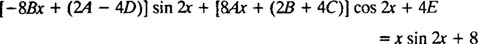In order for this last equation to be an identity, ABCD, and E must be chosen so thatThese equations determine the coefficients: A = 0, B = −⅛, C =D = 0, and E = 2. Therefore, a particular solution of the given differential equation is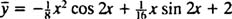According to Theorem B, then, combining this y with y h gives the complete solution of the nonhomogeneous differential equation: